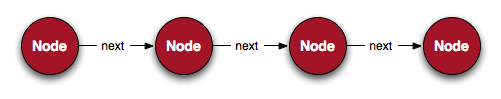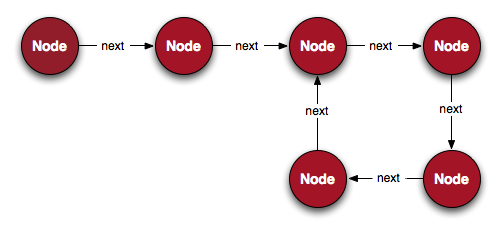## Interview Questions: Loops in Linked Lists

by Jesse Farmer on Wednesday, May 14, 2008

This is part of my series on interview questions, so welcome aboard!

This installment deals with a common question about linked lists — how do we detect when one has a loop?

Linked lists are one of the most simple data structures and most aspiring programmers learn them early on. But for completeness' sake let's cover that ground.

A linked list is a sequence of nodes. Each node contains a piece of data and a reference to the next node in the list. Graphically it looks like thisIt's possible, though, that a node in a linked list might point to a previous element in the list. This is bad for many reasons, not the least of which is that any loop which iterates over all the nodes in the list by accessing the next node will never terminate.

So, it becomes important to detect when linked lists have loops. Here's what one such errant linked list looks like.### The Easy Solution

The easy solution is to keep track of every node seen so far and check if the current node is in that list. Here's a very simple linked list implementation in Ruby.

```class Node
attr_accessor :data, :next

def initialize(data = nil)
@data = data
@next = nil
end
end```

Here is the simple solution for detecting loops using the above implementation

```def has_loop?(node)
seen = []
until node.next.nil? do
return true if seen.include? node
seen << node
node = node.next
end
false
end```

This solution is workable but sub-optimal (surprise!). This has O(n2) complexity in CPU and O(n) complexity in memory, but a solution with O(n) complexity in CPU and O(1) complexity in memory is possible. In fact, this question is usually posed to preclude the above solution.

### The Tortoise and the Hare

The better solution involves a bit of mathematical thinking. If there is a loop then that means any iterator, no matter how many steps it takes per iteration, must hit the offending node.

So, if we have two iterators, one of which has a length that is a multiple of the other, they'll eventually land on the same node. The usual solution is to have one iterator advance one at a time ("the tortoise") and a second iterator advance two at a time ("the hare").

That algorithm looks like this

```def has_loop?(node)
slow = node
fast = node
until slow.next.nil? or fast.next.nil? do
slow = slow.next
fast = fast.next.next
return true if (slow == fast)
end
false
end```

### More Questions

Assuming you answer the above correctly and quickly the interviewer will probably follow up with some related questions. How do you fix the linked list when you detect a loop? What is the linked list has multiple loops? How do you determine the size of the loop?

I'll leave you to ponder these questions. Cheers!